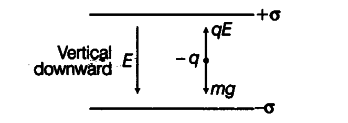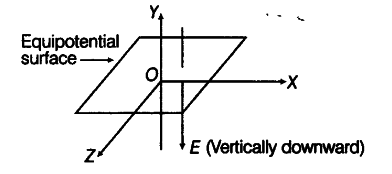# Two uniformly large parallel thin plates having charge densities + $\sigma$ and - σ

Two uniformly large parallel thin plates having charge densities + $\sigma$ and - σ are kept in the XZ-plane at a distance d apart. Sketch an equipotential surface due to electric field between the plates. If a particle of mass m and charge - q remains stationary between the plates. What is the magnitude and direction of this field?

Here, — q charge experiences force in a direction opposite to the direction of electric field.-q charge balances, when
qE = mg ====> E = mg/q

The direction of electric field is along vertically downward direction.

The skectch of equipotential surface due to electric field between the plates is shown in figure below.